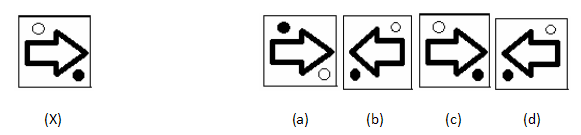# Image Analysis - Non Verbal Reasoning questions

## Image Analysis - Non Verbal Reasoning questions

1. Find out which of the figure (a), (b), (c), (d) can be formed from the pieces given in fig. (X).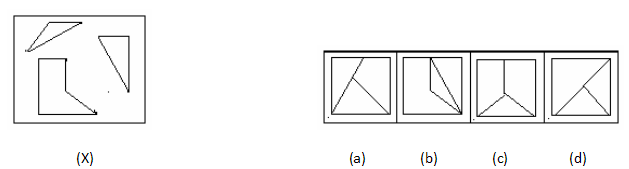2. Find out which of the figure (a), (b), (c), (d) can be formed from the pieces given in fig. (X).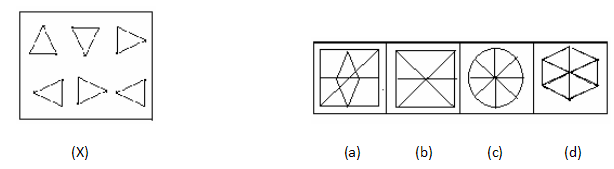3 Find out which of the figure (a), (b), (c), (d) can be formed from the pieces given in fig. (X).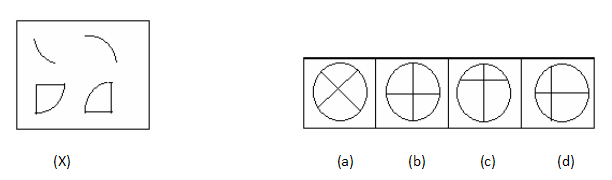4. Find out which of the figure (a), (b), (c), (d) can be formed from the pieces given in fig. (X).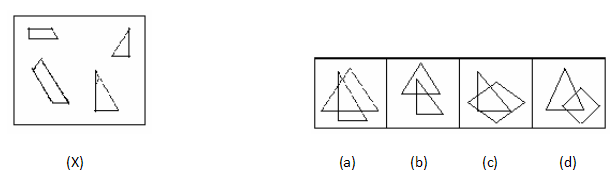5 Find out which of the figure (a), (b), (c), (d) can be formed from the pieces given in fig. (X).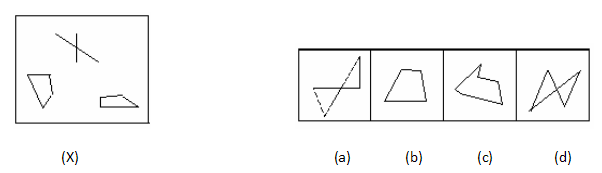6 Find out which of the figure (a), (b), (c), (d) can be formed from the pieces given in fig. (X).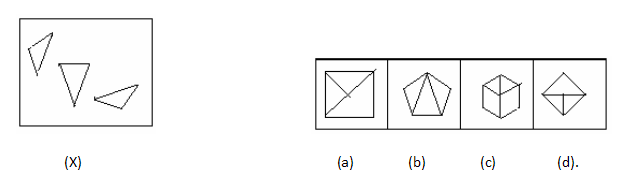7. Find out which of the figure (a), (b), (c), (d) can be formed from the pieces given in fig. (X).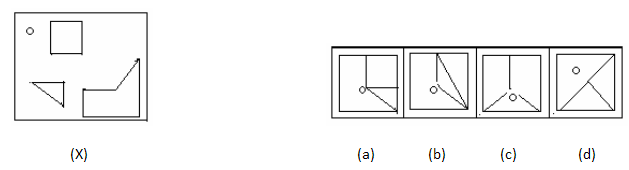8. Find out which of the figure (a), (b), (c), (d) can be formed from the pieces given in fig. (X).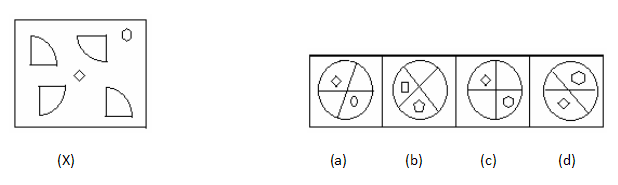9 . Find out which of the figure (a), (b), (c), (d) can be formed from the pieces given in fig. (X).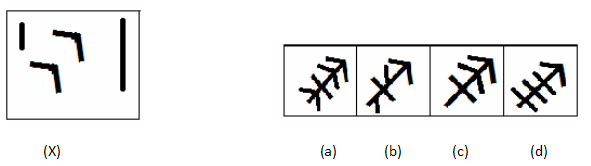10. Find out which of the figure (a), (b), (c), (d) can be formed from the pieces given in fig. (X).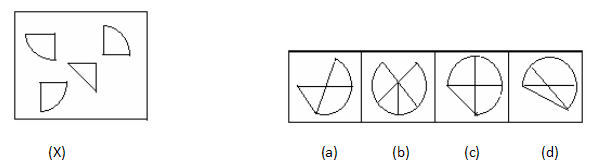11. Find out which of the figure (a), (b), (c), (d) can be formed from the pieces given in fig. (X).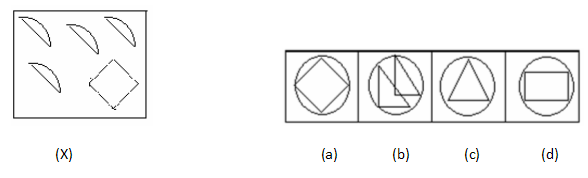12. Find out which of the figure (a), (b), (c), (d) can be formed from the pieces given in fig. (X).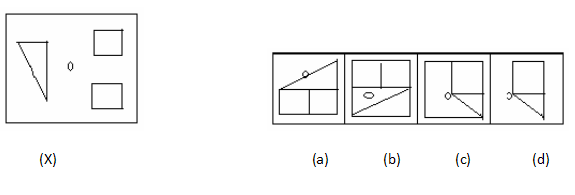13. Find out how the key figure (X) will look like after rotation.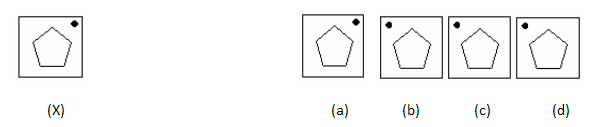14. Find out how the key figure (X) will look like after rotation.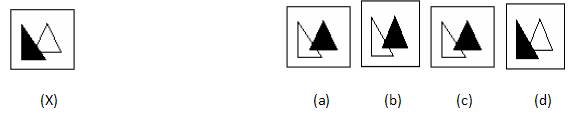15. Find out how the key figure (X) will look like after rotation.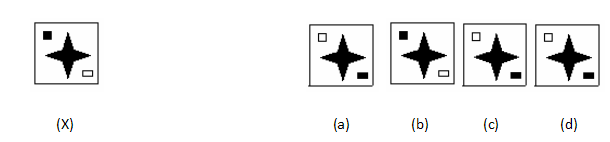16. Find out how the key figure (X) will look like after rotation.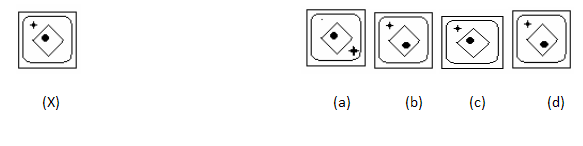17. Find out how the key figure (X) will look like after rotation.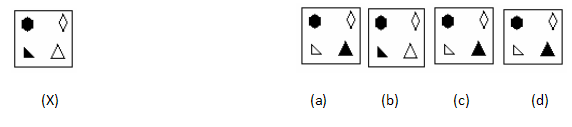18. Find out how the key figure (X) will look like after rotation.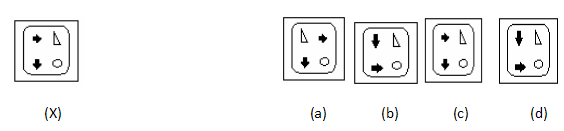19. Find out how the key figure (X) will look like after rotation.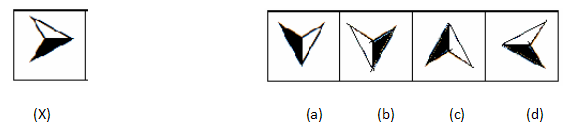20. Find out how the key figure (X) will look like after rotation.